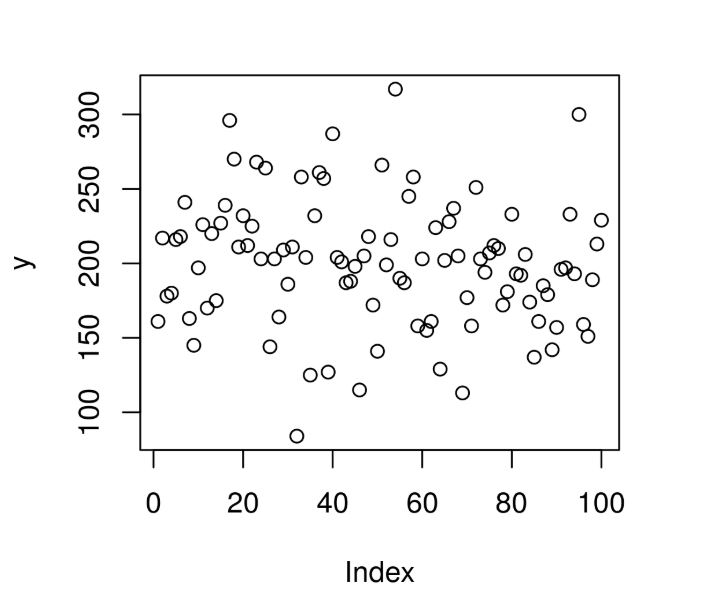GeeksforGeeks App
Open AppBrowser
Continue

# Compute Randomly Drawn Wilcoxon Rank Sum Density in R Programming – rwilcox() Function

`rwilcox()` function in R Language is used to compute random density for Wilcoxon Rank Sum Statistic Distribution over a sequence of random Numeric values.

Syntax: rwilcox(N, m, n)

Parameters:
N: Size of Random Vector
m: Larger sample size
n: Smaller sample size

Example 1:

 `# R Program to compute random``# Wilcoxonrank Sum Density`` ` `# Setting seed for``# random number generation``set``.seed(``1000``)`` ` `# Set Vector size``N <``-` `20`` ` `# Calling rwilcox() Function``y <``-` `rwilcox(N, m ``=` `10``, n ``=` `5``)``y`

Output:

```  18 21 30 32 12 32 21 24 33 24 26 35 18 27 29 21 13 15 26 31
```

Example 2:

 `# R Program to compute random``# Wilcoxonrank Sum Density`` ` `# Setting seed for``# random number generation``set``.seed(``1000``)`` ` `# Set Vector size``N <``-` `100`` ` `# Calling rwilcox() Function``y <``-` `rwilcox(N, m ``=` `40``, n ``=` `10``)`` ` `# Plot a graph``plot(y)`

Output:My Personal Notes arrow_drop_up